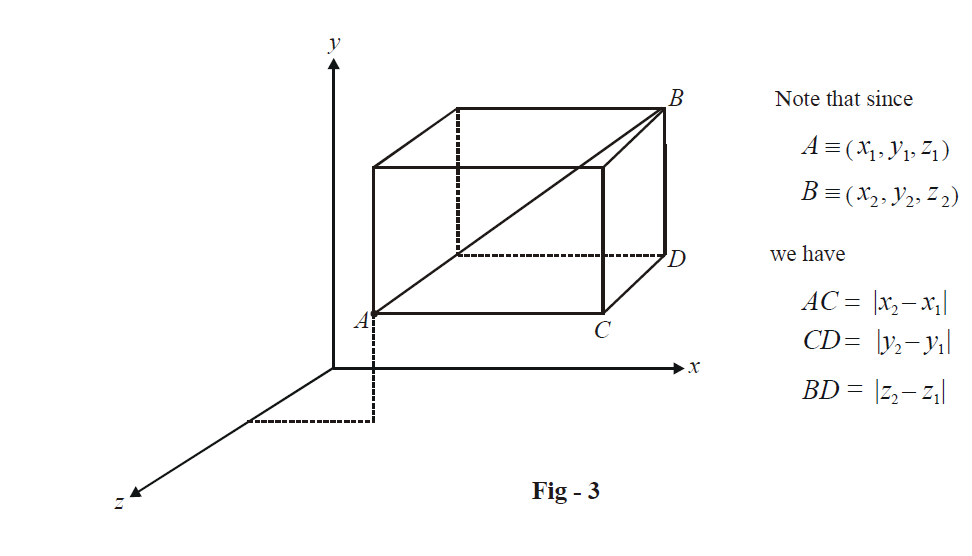# Three Dimensional Distance Formula

Go back to  'Three Dimensional Geometry'

## DISTANCE FORMULA

Let  $$A({x_1},\,{y_1},\,{z_1})$$  and   $$B({x_2},\,{y_2},{z_2})$$  be two arbitrary points. We need to find the distance between A and B in terms of the coordinates of A and B. Observe the following diagram carefully:AB is simply the length of the diagonal of the cuboid drawn (as depicted above):

\begin{align}&\quad \;\;\;\;\;A{B^2} = A{C^2} + C{D^2} + B{D^2} \\\\&\quad \;\;\;\;\;A{B^2} = {\left( {{x_1} - {x_2}} \right)^2} + {\left( {{y_1} - {y_2}} \right)^2} + {\left( {{z_1} - {z_2}} \right)^2} \\\\ &\Rightarrow\quad \boxed{AB = \sqrt {{{\left( {{x_1} - {x_2}} \right)}^2} + {{\left( {{y_1} - {y_2}} \right)}^2} + {{\left( {{z_1} - {z_2}} \right)}^2}} } \\ \end{align}

This is the distance formula for three dimensions. It has a form exactly similar to the distance formula for two dimensions.

As an example, let  $$A \equiv \left( {1,\,\,2,\,\,3} \right),\,\,B \equiv \left( {3,\,\,2,\,\,1} \right)$$  and   $$C \equiv \left( {2,\,\,2,\,\,2} \right).$$  We have,

\begin{align}&AB = \sqrt {{{\left( {1 - 3} \right)}^2} + {{\left( {2 - 2} \right)}^2} + {{\left( {3 - 1} \right)}^2}} = 2\sqrt 2 \\\\ & BC = \sqrt {{{\left( {3 - 2} \right)}^2} + {{\left( {2 - 2} \right)}^2} + {{\left( {1 - 2} \right)}^2}} = \sqrt 2 \\\\ &AC = \sqrt {{{\left( {1 - 2} \right)}^2} + {{\left( {2 - 2} \right)}^2} + {{\left( {3 - 2} \right)}^2}} = \sqrt 2 \\ \end{align}

Incidentally, C is the mid point of AB since AC + BC = AB and AC = BC.

Example – 1

Find the locus of the point P equidistant from the four points  $$O\left( {0,\,0,\,0} \right),\,\,A\left( {x,\,0,\,0} \right),\,B\left( {0,\,y,\,0} \right)$$ and  $$C\left( {0,\,0,\,z} \right).$$

Solution: Let  $$P \equiv \left( {a,\,b,\,c} \right)$$

We have,

\begin{align}&\quad \;\;\;\;\;O{P^2} = A{P^2} \\\\ &\Rightarrow\quad {a^2} + {b^2} + {c^2} = {\left( {a - x} \right)^2} + {b^2} + {c^2} \\ \\ &\Rightarrow\quad {a^2} = {\left( {a - x} \right)^2} \\\\ \,\,\,\,\,\,\,\,\,\,\,\,\,\,\,\,\,\,\,\,\,\, &\qquad\quad\;= {a^2} + {x^2} - 2ax \\\\ &\Rightarrow\quad a = \frac{x}{2} \\ \end{align}

Similarly, \begin{align}b = \frac{y}{2}\end{align} and  \begin{align}c = \frac{z}{2}.\end{align} Thus, the point P is \begin{align}\left( {\frac{x}{2},\frac{y}{2},\frac{z}{2}} \right).\end{align}

Example – 2

Find the locus of a point P which moves so that its distances from the points A (0, 2, 3) and B ( 2, –2, 1) are always equal.

Solution:P will obviously lie on the perpendicular bisector of AB. Let the co-ordinates of P be (x, y, z). Therefore,

\begin{align}&\qquad\;\; P{A^2} = P{B^2} \\\\ &\Rightarrow\quad {x^2} + {\left( {y - 2} \right)^2} + {\left( {z - 3} \right)^2} = {\left( {x - 2} \right)^2} + {\left( {y + 2} \right)^2} + {\left( {z - 1} \right)^2} \\\\ &\Rightarrow \quad x - 2y - z + 1 = 0 \\\end{align}

This is the required locus of P (if you think carefully, it is a plane)

Learn from the best math teachers and top your exams

• Live one on one classroom and doubt clearing
• Practice worksheets in and after class for conceptual clarity
• Personalized curriculum to keep up with school